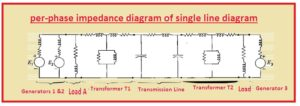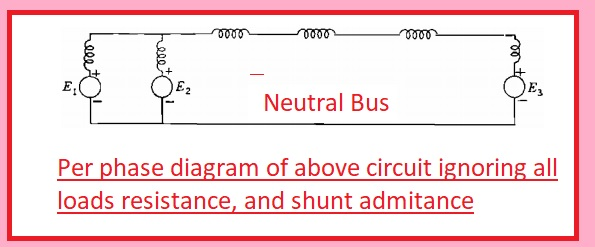Hello readers in this post we will discuss Impedance and Reactance Diagrams Of Electrical System There is a basic and easy way is used to drawn and explain the single line diagram in power system through use of impedance and reactance diagrams.

This way helps to study the power flow and complicated measurement of the system. As there is power calculation is somewhat difficult to process in the three-phase system and measurement are easy through the use of these diagrams for an unbalanced and unsymmetrical network.

For this complicated network, there is the usage of an impdance and reactance diagram to make the system simple and easy to understand. Here we learn different parameters that will help us to understand the power system easily and single line diagram.SO let get started

## Impedance And Reactance Diagrams Of Electrical System

• For measurements of operation of the system in case of the load is connected or there is a certain fault is exist in the system there is ingle line diagram is used for making the per phase system or single phase of the circuitry.
• There is resultant circuitry of the system shown in the above figure is created that is per phase impedance diagram.• If we do a load study of the system then lagging load denoted with A and B are shown through resistive and inductance reactance linked in series combination.
• At the impedance diagram, there is no display of limiting impedance denoted at the single line diagram linked among the neutral wire of the generator and ground terminal.
• It is due to that there is no current in-ground and neutral in case of the balance system.
• SHunt current has no importance as compare to full load current so shunt admittance is not added in the circuitry.
• For fault calculation, there is no existence of resistance.
• resistance and inductive reactance are not added.
• Loads that are not included rotating devices like motors have less effect at the total line current in case of the fault so it is not added to the diagram.
• During faults measures, synchronous motor is added because their output volts are working in case of short circuitry current.
• The digram should consider induction motor in consideration through generated volts in series to the inductive reactance it occurs in the case when it using for calculation of current in when fault exist the system.
• There is no use of induction motor in the circuit in case of current measures since after occurrence of fault current delivering through induction motor will be eliminated when it becomes a short circuit.
• If we want to make simple of our calculation for measurements of current through ignoring all static loads like a transformer, , shunt admittance, transmission line capacitance, the resistance of system then impedance diagram will become per phase diagram shown below